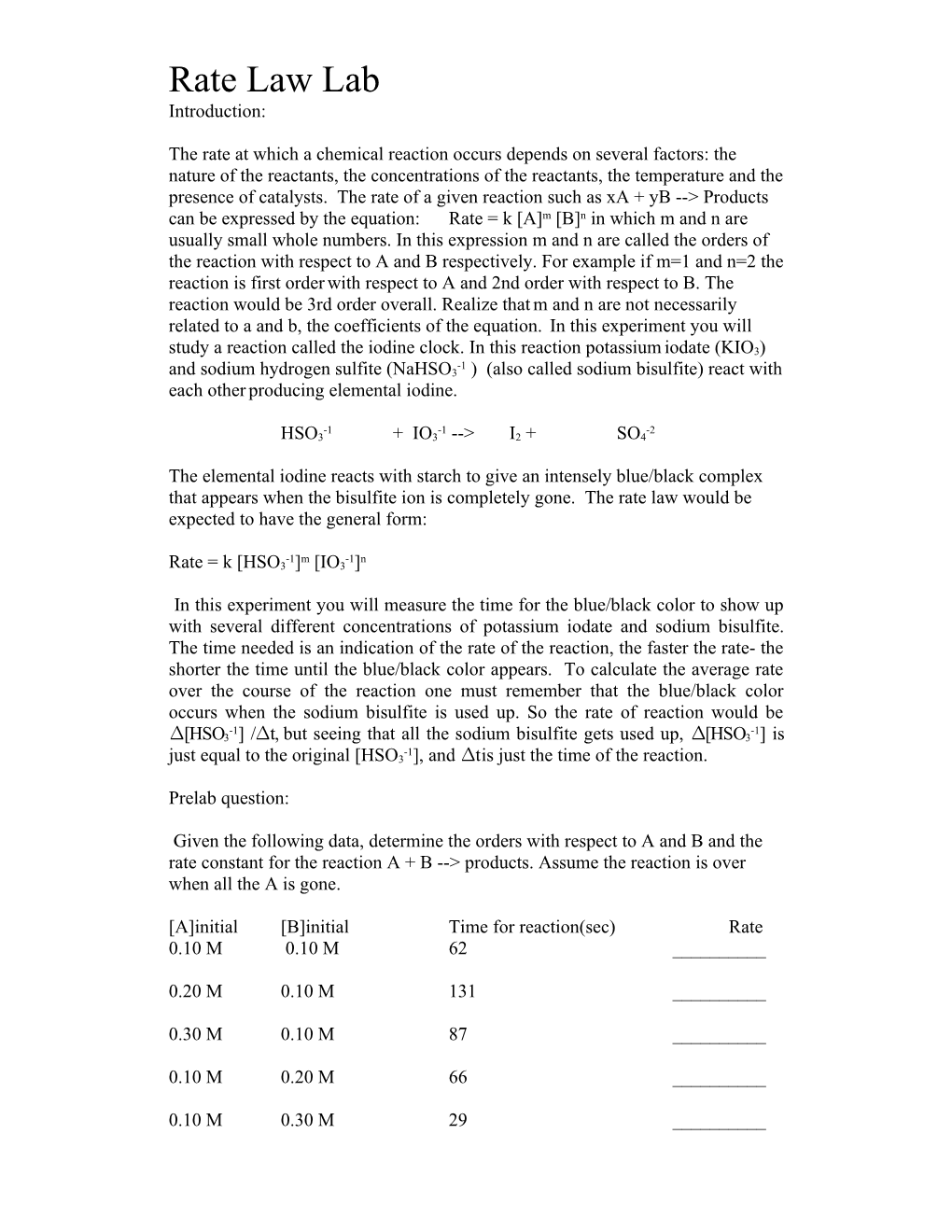# The Rate at Which a Chemical Reaction Occurs Depends on Several Factors: the Nature OfRate Law Lab

Introduction:

The rate at which a chemical reaction occurs depends on several factors: the nature of thereactants, the concentrations of the reactants, the temperature and the presence of catalysts. The rate of a given reaction such as xA + yB --> Products can be expressed by the equation: Rate = k [A]m[B]n in which m and n are usually small whole numbers. In this expression m and n are called the orders ofthe reaction with respect to A and B respectively. For example if m=1 and n=2 the reaction is first orderwith respect to A and 2nd order with respect to B. The reaction would be 3rd order overall. Realize thatm and n are not necessarily related to a and b, the coefficients of the equation. In this experiment you will study a reaction called the iodine clock. In this reaction potassiumiodate (KIO3) and sodium hydrogen sulfite (NaHSO3-1 ) (also called sodium bisulfite) react with each otherproducing elemental iodine.

HSO3-1+ IO3-1--> I2+ SO4-2

The elemental iodine reacts with starch to give an intensely blue/black complex that appears when the bisulfite ion is completely gone. The rate law would be expected to have the general form:

Rate = k [HSO3-1]m[IO3-1]n

In this experiment you will measure the time for the blue/black color to show up with several different concentrations of potassium iodate and sodium bisulfite. The time needed is an indication of the rate of the reaction, the faster the rate- the shorter the time until the blue/black color appears. To calculate the average rate over the course of the reaction one must remember that the blue/black color occurs when the sodium bisulfite is used up. So the rate of reaction would be ∆[HSO3-1] /∆t, but seeing that all the sodium bisulfite gets used up, ∆[HSO3-1] is just equal to the original [HSO3-1], and ∆t is just the time of the reaction.

Prelab question:

Given the following data, determine the orders with respect to A and B and the rate constant for the reaction A + B --> products. Assume the reaction is over when all the A is gone.

[A]initial [B]initial Time for reaction(sec)Rate

0.10 M 0.10 M 62______

0.20 M 0.10 M 131______

0.30 M 0.10 M 87 ______

0.10 M 0.20 M 66______

0.10 M 0.30 M 29______

Procedure:

Three solutions have been prepared for your use in lab. Solution A is 0.030 M potassium iodate, solution B is 0.2% starch in distilled water and solution C is 0.015 M sodium bisulfite. You will be mixing an amount of A, B and C together and measuring the time needed for the blue/black color to appear. Youwill have to use the dilution equation to calculate the concentration of iodate and bisulfite in each of the seven solutions. For each reaction # run the reaction two times and use the average of the two times if they are within 10% of each other.

Rctn.mL [IO3-] mL Starch mL [HSO3-]Time 1Time 2Avg. Time

1 5.0 15.0 5.0______

2 5.0 12.5 7.5______

3 5.0 10.0 10.0______

4 5.0 7.5 12.5______

5 7.5 12.5 5.0______

6 10.0 10.0 5.0______

7 12.5 7.5 5.0______

Mix the starch solution and whichever solution is not 5.0 mL in a 50 mL flask, inject the the 5.0 mL solution and start the stopwatch, agitating the solution every 30 seconds or so to make sure it is mixed. Do not agitate too vigorously as bisulfite is oxidized by the oxygen in the air. Make sure to label a pipette for each of the reactants, and rinse the flask between runs with distilled water.

Write-up:

1. Show the calculations necessary to determine the initial concentrations of each reactant for EACH TRIAL

2. Assuming that the bisulfate ion is the limiting reactant, calculate the rate for EACH TRIAL.

3. Use the method of initial rates to determine m and n for the reaction we have done. Show all your calculations for one determination of each m and n.

4. After determining m and n, use each of the data points to determine a value of k for EACH TRIAL and average the values to get a final value of k. Give a final equation for the reaction including m, n and k.

5. assume that the solution is in an acidic environment and use redox technique to balance the equation.

Construct a plot of [iodate] (x-axis) vs. rate (y-axis) using points 1,5,6 &7. Make another plot of [bisulfite] (x-axis) vs. rate (y-axis) using points 1,2,3 &4. If these are linear the reaction is first order with respect to that reactant. If one is not straight, try graphing [concentration]2 vs rate to see if it is second order, [concentration]3 if it is third order, [concentration]1/2 if it is one-half order. Include all graphs in your write up.

The reaction mechanism……..